# RNN Simplified- A beginner’s guide

Source: Deep Learning on Medium

## An intuitive explanation of the Recurrent Neural Network , LSTM and GRU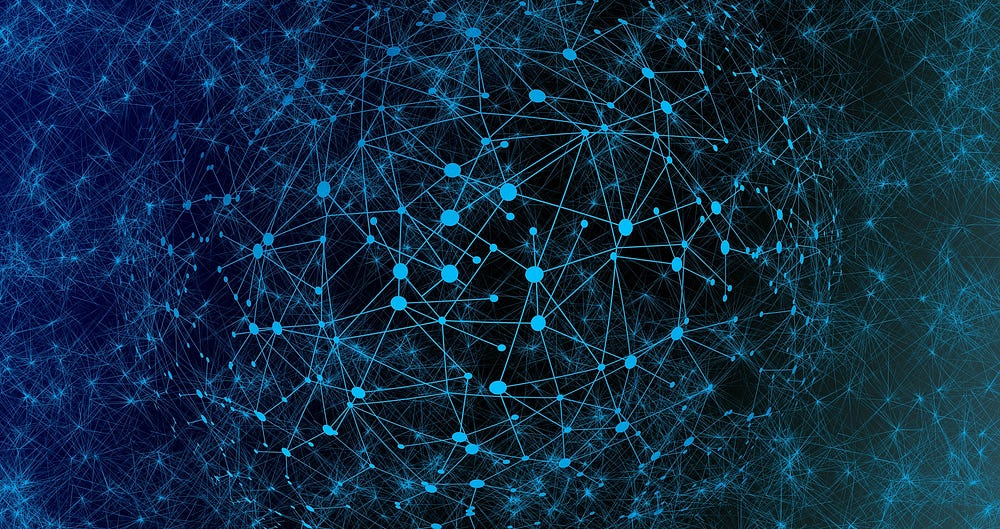image credit — Gerd Altmann from Pixabay

Recurrent Neural Network(RNN) is a popular architecture of Neural Network which is used extensively with use cases consist of sequential or contextual data.

Before we start with the RNN itself let’s first see why we need it in the first place.Let’s try to remember this scene.

It took a while, right?(or may be you are not able to relate at all)

Now imagine that you are watching the movie and you are in this particular scene , you will able to connect the dots very easily. All our thinking goes in a flow and based on previous frame we can connect the current frame easily.Our thoughts have persistence.

Our traditional neural network architectures cannot do this, given a particular sequence of frames they cannot predict what is happening at each point in the movie.

To solve this kind of problem we use a network with a self loop.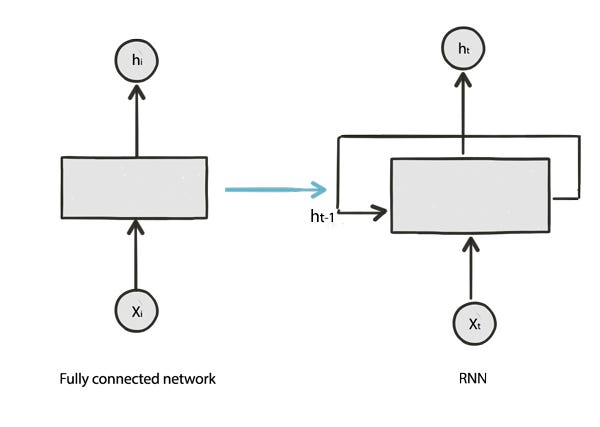fig1 : Recurrent Neural Network ( Network with self loop)

Simply,we feed back the output of the previous time frame to the next time frame in the network .Suppose the output of the network at t=1 is h0,while training the network at t=2 we will also consider h0,the output received from previous instance of time. If we unroll the network we will get the below structure.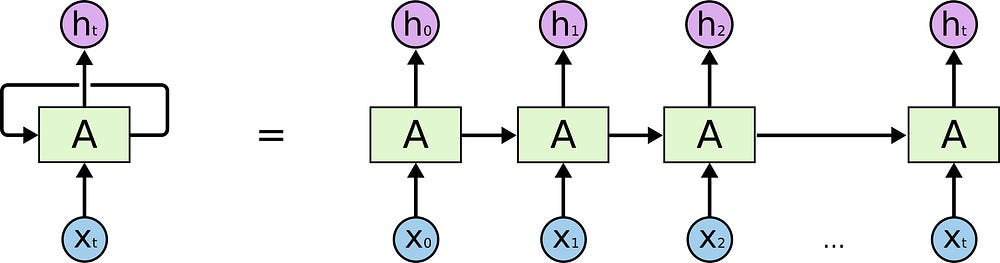fig2 : RNN at various instance of time , Image Credit— colah’s blog

The important point to remember here is that the sequential units you are showing are the same unit at different point of time and are not cascading units.

Now there are problems with the simple implementation of RNN too. They learn through back propagation over time.This could lead to vanishing gradient or exploding gradient problems if we ask them to learn from long term dependencies.In simple words they can not remember important information that may require in a later time stamp.

To overcome these problems we use LSTM (long short term memory),a very special kind of recurrent network and GRU (Gated Recurrent Unit) which is a slightly modified version of LSTM.

#### Breakdown of LSTM :

Let’s break down the internal structure of LSTM. A LSTM unit mainly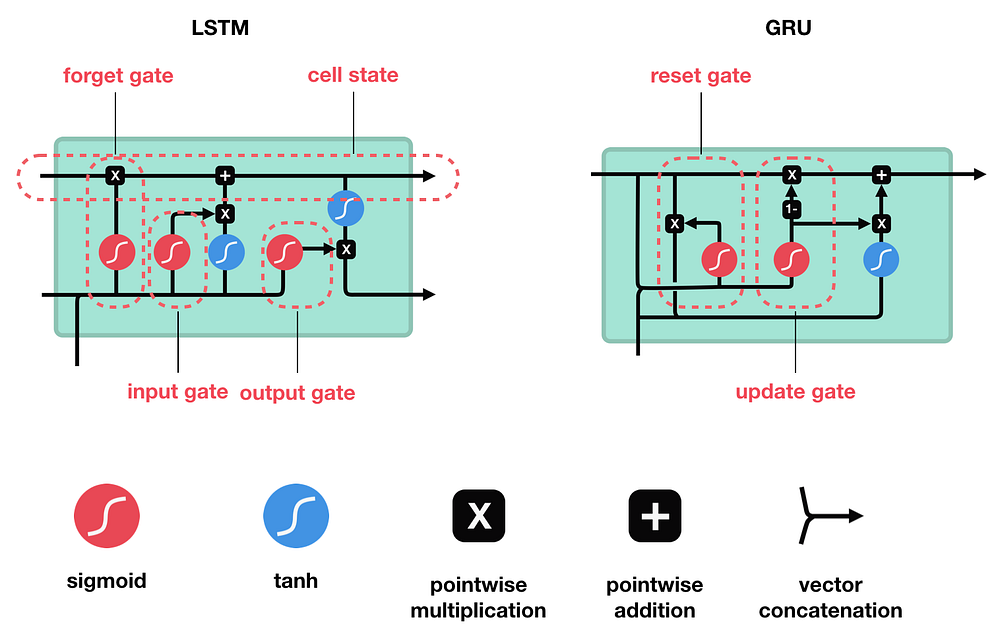fig3 :LSTM and GRU .image credit — Michael Nguyen

consists of a cell state(current information flow of the unit) and three Gates (read a special formation of network layers)- forget gate,input gate and output gate.Confusing right? Don’t worry we it break it down step by step.

Cell State: We can imagine cell state as the continuous flow of information over various instances of time. At each instance of time we have to decide how much information we will retain or modify.remember why we needed the LSTM in the first place ?we were not able to retain the importance of the information that comes from a particular instance of time far away. Here we have the flexibility to decide which information we will give more importance at each stage.

1. Forget gate: first let’s take a closer look at the various notations we have :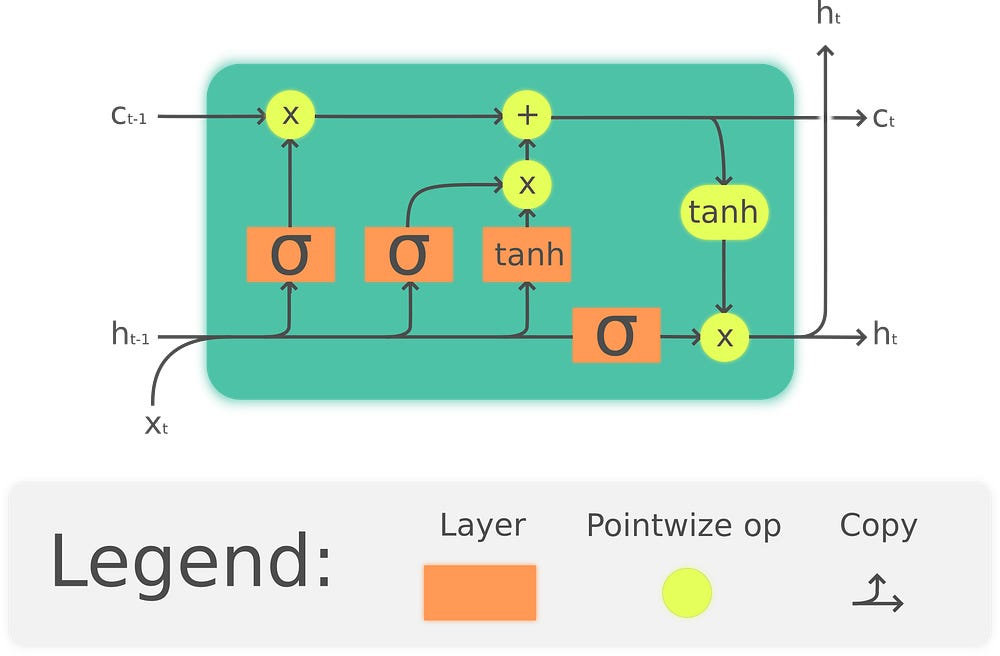fig4 :Structure of LSTM ,image credit- wikipedia

C_(t-1) : old cell state ,c_t: current cell state,h_(t-1):output from the previous state,h_t= output of the current state

Forget gate decides how much information we will use from the previous cell state and how much we will ‘throw away.’ The output from last state (h_(t-1)) is concatenated(not added) with x_t and passed through a sigmoid unit. Sigmoid provides output between 0 and 1. Intuitively 0 means ‘Forget everything’ and 1 means ‘Retain everything’.

2. Input gate: The input gate decides which new information we are going to add to the cell state. The concatenated x_t and h_(t-1) is sent over a sigmoid unit which decides what value we will update.

The concatenated value is also passed through a tanh layer which gives an output between -1 to +1 and helps to regulate the network.we then multiply the tanh output with sigmoid output and add with the cell state. After all of these operation we get the current value of our cell state.

3. Output gate: The output gate decides what information we will pass to the network in the next instance of time.If you follow the last horizontal line, we first concatenated x_t and h_(t-1) is sent over a sigmoid unit.Then we pass the value of current cell state through a tanh function (Note this is a point wise tanh operation,not a tanh activation layer).Finally we multiply both of the outputs and sent over the next instance of time. A question may come to your mind that why we are getting two h_t here ?.The answer is throughout our explanation we are considering a single unit of LSTM.In practice we can also use multiple layer of LSTMs which are cascaded.So one output goes to the next layer of the network and other goes to the next instance of network (feed forward with time).

#### Break down of GRU:

Gated Recurrent Unit is a popular variant of LSTM network introduced by

Cho, et al. (2014) . The main difference between LSTM and GRU is, GRU has only two gates (update and reset gate).

1. Update gate : A update gate is nothing but the combination of input and forget gate in a single unit .it decides what information to retain and what new information need to be added.
2. Reset gate : It decides what information needs to be passed to the network in the next instance of time. It also merges the current cell state and hidden state, and makes some other changes.

GRU has a reduced number of gates hence it reduces number of tensor operations compare to LSTM .This makes it computationally cheaper and faster than LSTMs. But we can not say which implementation is best straight away. It depends on the problem at hand.In practice we apply both models to our use case and compare which performs better.